Home MathLab Matlab Matrix Operations

# Matlab Matrix Operations

If you ever tried to work with huge matrices, you will know how unpleasant and tedious. Here is where Matlab come to play, it makes working with Matrices easier.

With Matlab, one of the major problem for beginners is to understand how the software works and what the software need in order to help them accomplish their goal using it.

In this read, we will hand over to you some basic Matlab Matrix operation and how to use them to get what you want.

Content:

## Matlab Matrix Operations

### Write a Matrix in Matlab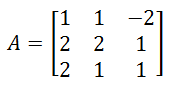we will write

`A=[1 1 -2;2 2 1;2 1 1]`

after pressing ENTER, here is how it will look in Matlab window

###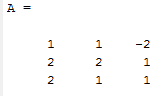Find the size of a Matrix

The size of a Matrix is its number of rows and columns. To find the size of a Matrix, use the following code

`size(A)`

Note A here is the matrix we created in the previous step.

Here is its size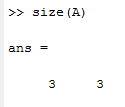Meaning, A has 3 rows and 3 columns.

Let’s try a second example.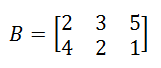If we type

`size(B)`

We will see the following

###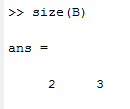Add Matrices

To add two matrices A and B, we need size(A) to be identical to size(B)

So, let’s create a new Matrix C with the same size as A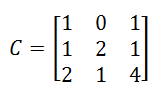Now we can add A and C using the following code

`A+C`

###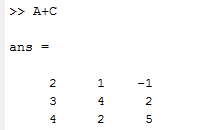Divide Matrices element by element

To divide two Matrices element by element use the following

`A./C`

Remember both matrices need to have the same size.

###Find the inverse of a Matrix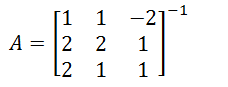To find the inverse of a Matrix, use the code:

`inv(A)`

###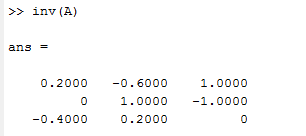Find the determinant of a Matrix

To find the determinant of a Matrix in Matlab, use the following code

`det(A)`

###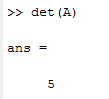Define a Matrix with Random elements

To create a Matrix with Random element in Matlab, use

`rand(3,2)`

Where (3,2) is the size of the Matrix

### Find the diagonal of a Matrix

DIAG help access diagonals of Matrices in Matlab.

To find the main diagonal of A, we will use

`diag(A)`

to find the first upper diagonal use

`diag(A,1)`

to find the first lower diagonal use

`diag(A,-1)`

Here is how Matlab read matrix diagonals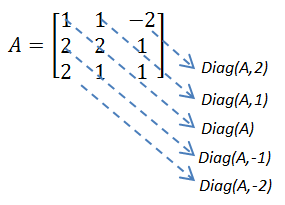### Compute the Transpose of a Matrix

To find the transpose of a Matrix, use the following

`A'`

or

`transpose(A)`

Here is the transpose of A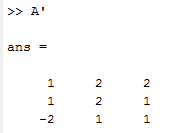### Extract an element in a Matrix

You can individually access element of a Matrix or a whole vector.

Let’s consider the following MatrixIf I need to access the first row of the Matrix, I will use the following code

`C(1,:)`

I will use the following to access the element on the first rows-second column.

`C(1,2)`

The following will help access element of the third column

`C(:,3)`

### Multiply Matrices

To multiply A X B, A and B being two distinct matrices, A and B has to obey these conditions.

To multiply A by B in Matlab, use the code

`A*B`

### Multiply 2 Matrices element by element

To multiply a Matrices element by element, remember the size of the two matrices has to be the same.

Use the following line

`A.*B`

### Create a Matrix with all elements equal to zero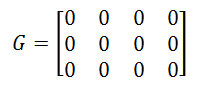To create a Matrix with all elements equal to zero, use the following code

`G=zeros(3,4)`

where (3,4) is the size of the Matrix

### Create a Matrix with All elements equal to one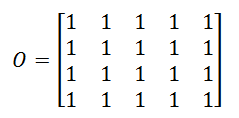To create a Matrix with all elements equal to one, use the following code

`O=ones(4,5)`

where (4,5) is the size of the Matrix

## Summary of Matrix functions

size: Size of a matrix
det: Determinant of a square matrix
inv: Inverse of a matrix
rank: Rank of a matrix
rref: Reduced row echelon form
eig: Eigenvalues
poly: Characteristic polynomial
norm: Norm of matrix
lu: LU factorization
svd: Singular value decomposition
eye: Identity matrix
zeros: Matrix of zeros
ones: Matrix of ones
diag: extract/create diagonal of a matrix
rand: randomly generated matrix

#### Related Posts

This website uses cookies to improve your experience. We'll assume you're ok with this, but you can opt-out if you wish. Accept Read More